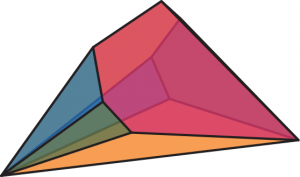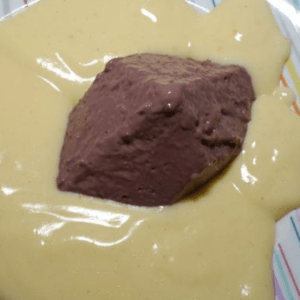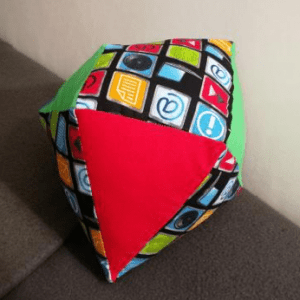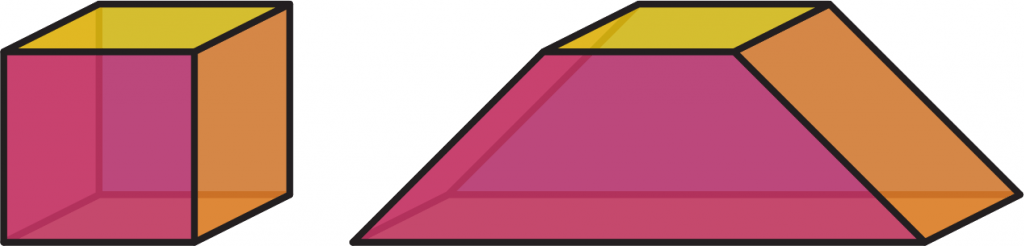Mara Kortenkamp, Erin Henning and Anna Maria Hartkopf give us a tour of Polytopia, a home for peculiar polytopesWould you like to have a mathematical object named after yourself? You no longer need to be Pythagoras to eternalise yourself in the terminology of mathematics.

#### A polyhedron is not just for ChristmasThis is Ecki. He lives in Polytopia.

Mathematicians currently know over a hundred trillion  different kinds of polyhedron and only a very small percentage of them actually have a name, like cube or pyramid. Not only does the cube possess a name and fame, it is also realised in gigantillions of models all over the world. But not for most polyhedra. These helpless shapes are nameless, unrealised, and lost in abstraction. You can help change that, today.

## Who are we?

Polytopia is a foster home for all polyhedra with up to nine vertices. We give them a home, regardless of their prominence or whether they’ve already got a name. When visiting Polytopia, you’ll be able to see all the polyhedra who have already found loving homes and, more importantly, meet the ones still waiting to be adopted.

#### But what can you do?

You can help us make a change and adopt one of these little geometrical creatures. By giving them a name and building a physical model of them, you can bring them into the real world. Visit us at Polytopia today and be inspired to rescue your new best friend from the realm of abstraction.One of the polyhedra that lives in Polytopia.

There are several definitions of polyhedra in mathematics. Let us start with talking about polygons, which you are probably already familiar with. A polygon is a two-dimensional shape, consisting of straight line segments, called edges, and points, called vertices, where two edges meet. The simplest example of a polygon is a triangle. One important type of polygon is the regular polygon: those which have edges of the same length, and with all internal angles the same. Examples of regular polygons are the equilateral triangle and the square.“I named my polyhedron Seems to be Piez and had a lot of fun crafting it.”“My polyhedron is called Oktavius and makes a lovely decoration for my room.”

From polygons we can naturally build up to polyhedra. A polyhedron is a geometrical object bounded by a finite number of polygons. In other words, a polyhedron is a three-dimensional object, whose faces are polygons, whose edges are the straight line segments where two such faces meet, and whose vertices are points where three or more faces meet.

One of the simplest (and most famous) polyhedra is the cube. It is built by glueing squares together and it consists of eight vertices connected by twelve edges and six faces.

In Polytopia, we are only interested in convex polyhedra, which means that all inner angles between two edges or two faces are less or equal to 180°. Thus, no indentations, holes or cavities are allowed, and all vertices and edges are ‘pointing outwards’.

The superstars of the polyhedral world are the Platonic solids. These five polyhedra, each consisting only of identical regular polygons, are named after the ancient Greek philosopher Plato. Starting with regular triangles, we can use them to build three of the five Platonic solids: the tetrahedron, the octahedron, and the icosahedron. Do you already know the two remaining Platonic solids? (Hint: one of them is the cube.)Three Platonic solids. Who’s missing?

Now you might ask yourself: how do we differentiate the polyhedra?“It was so much fun modelling my polyhedron Pullyeder out of chocolate ice cream, especially because I ate it afterwards!”“Since I was in need of a new pillow, I decided to sew my polyhedron Flori into one.”

We at Polytopia distinguish polyhedra by their combinatorial type and not their geometric realisation. The combinatorial type describes the structure of the vertices, edges, and faces of a polyhedron but it is not sensitive to the size or even the shape of a polyhedron. Given two polyhedra, take out the faces as if they were made of glass. What remains is the frame of the edges. Now imagine that you can mould and transform this frame in any way you like, but you cannot disconnect any of the edges or vertices. If you can transform the frame of one polyhedron into the other without making or breaking any connections, then these two polyhedra are considered combinatorially equivalent.

The two pictures on the next page show the cube but in different geometric realisations. The one on the left looks like what you might expect and the one on the right was generated by modifying the size and shape of the usual cube. But notice that the vertices can still be matched to one another, preserving the edges’ connections. Therefore, they are combinatorially equivalent, and are both called Cube.Cube standing up straight, and Cube slouching.

Unfortunately, even with combinatorial equivalence, there are so many different polyhedra that it is infeasible for mathematicians to name them all. That’s why we here at Polytopia invite you to name as many polyhedra as you can. Every little helps.

 114,683,158,574,357 to be precise. This is the number of combinatorial types of polyhedron with up to 18 vertices. No mathematician has been able to give the number for 19 vertices exactly: so far for more than 18 vertices, only upper bounds on the number of combinatorial types are known. Even the computers we have today would take forever to construct and count all types for 19 vertices.Mara is studying computer science, and is working as a student assistant within the discrete geometry workgroup at Freie Universität Berlin.Erin is studying mathematics, and is working as a student assistant within the discrete geometry workgroup at Freie Universität Berlin.Anna Maria is a professional maths communicator and is currently writing her PhD about her project, Polytopia.